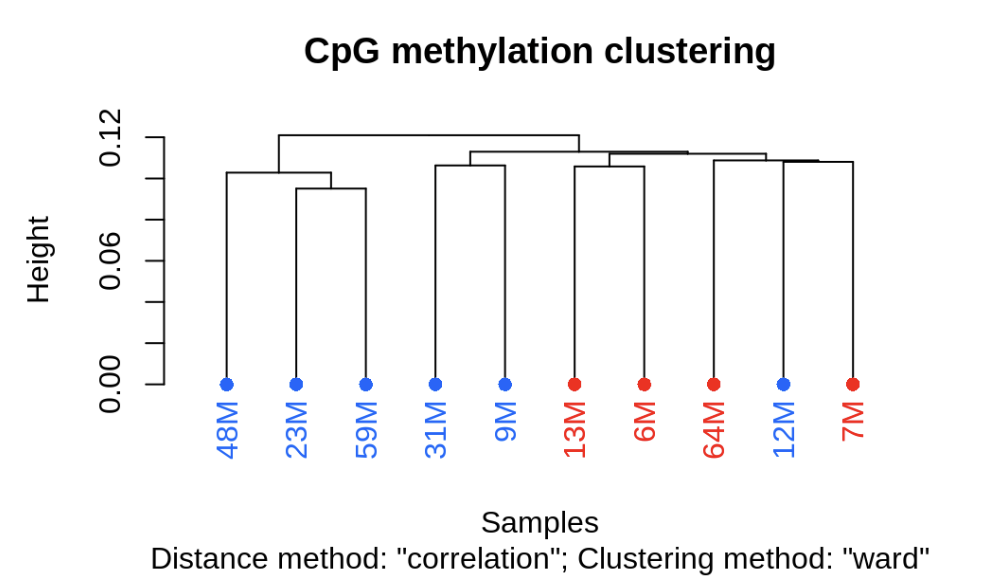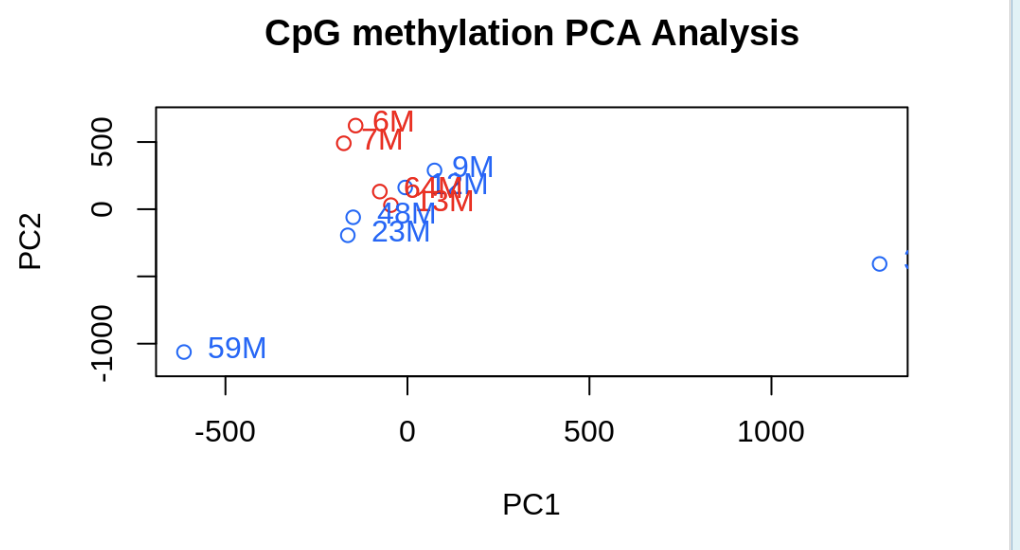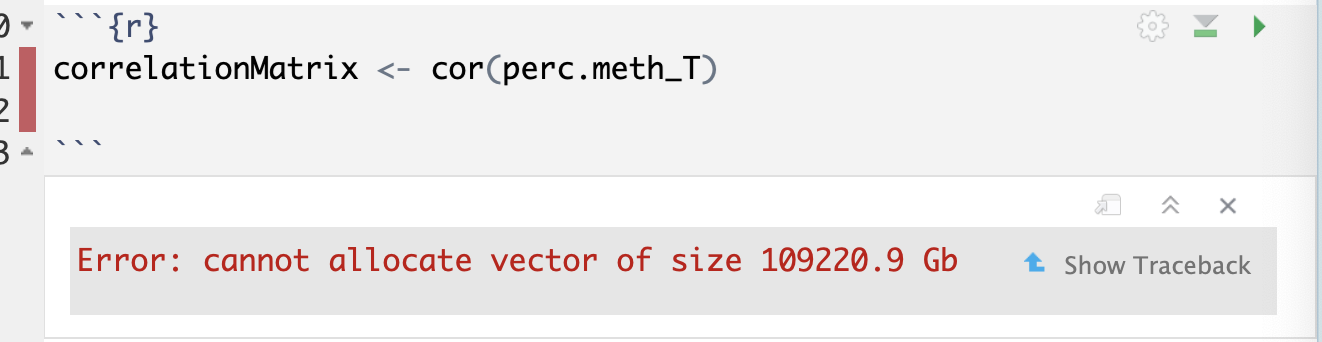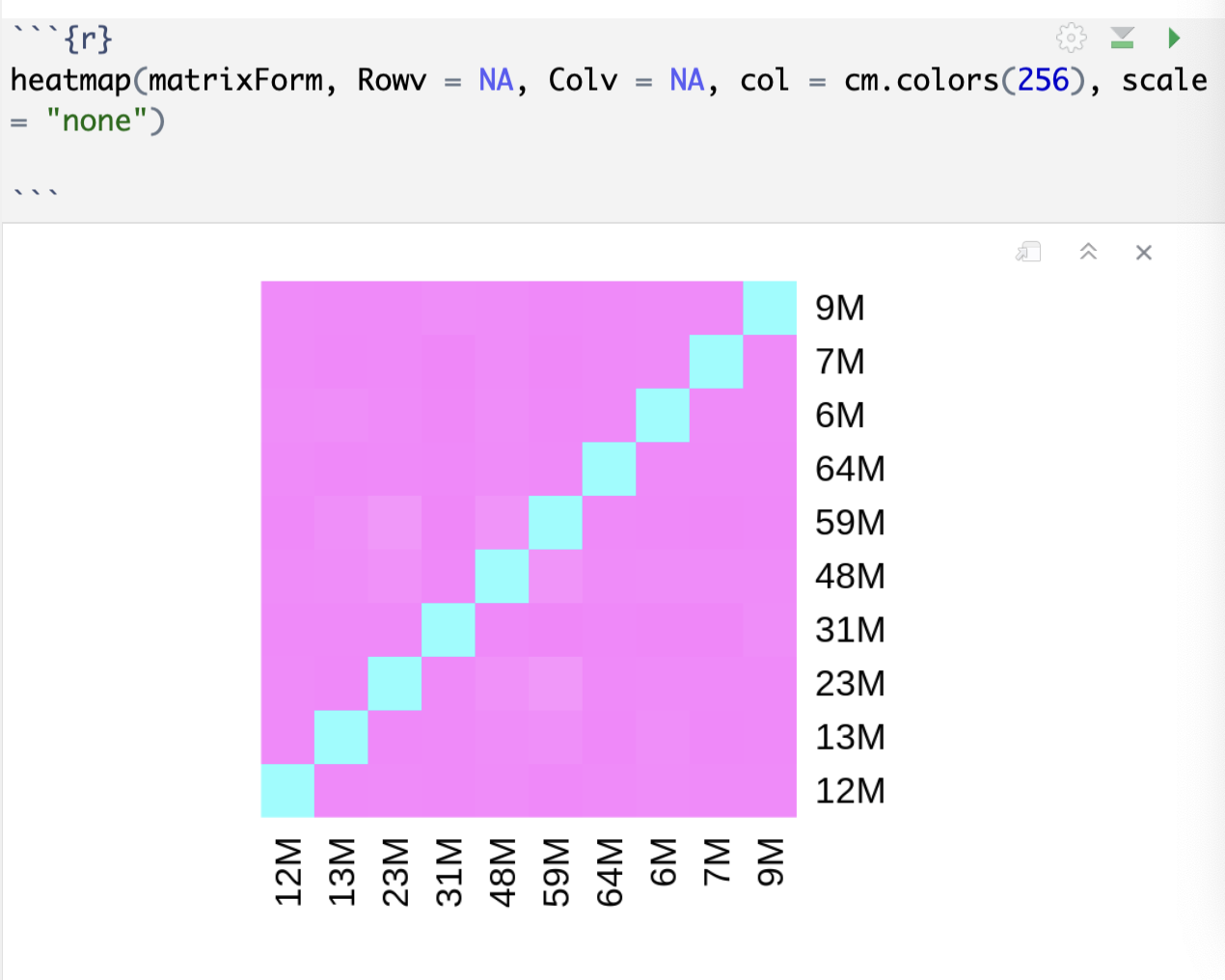# methylation distance matrix

Here is an attempt to pull relatedness / distance matrix from methylkit data…

``````library(tidyverse)
library(methylKit)
``````

Sample.ID OldSample.ID Treatment Sex TreatmentN Parent.ID
12M S12M Exposed M 3 EM05
13M S13M Control M 1 CM04
16F S16F Control F 2 CF05
19F S19F Control F 2 CF08
22F S22F Exposed F 4 EF02
23M S23M Exposed M 3 EM04
29F S29F Exposed F 4 EF07
31M S31M Exposed M 3 EM06
35F S35F Exposed F 4 EF08
36F S36F Exposed F 4 EF05
39F S39F Control F 2 CF06
3F S3F Exposed F 4 EF06
41F S41F Exposed F 4 EF03
44F S44F Control F 2 CF03
48M S48M Exposed M 3 EM03
50F S50F Exposed F 4 EF01
52F S52F Control F 2 CF07
53F S53F Control F 2 CF02
54F S54F Control F 2 CF01
59M S59M Exposed M 3 EM01
64M S64M Control M 1 CM05
6M S6M Control M 1 CM02
76F S76F Control F 2 CF04
77F S77F Exposed F 4 EF04
7M S7M Control M 1 CM01
9M S9M Exposed M 3 EM02
``````myobj_m = processBismarkAln(location = file.list_male,
sample.id = list("12M","13M","23M","31M","48M","59M","64M","6M","7M","9M"),
assembly = "cv",
mincov=2,
treatment =  c(1,0,1,1,1,1,0,0,0,1))
``````
``````save(myobj_m, file = "../analyses/myobj_m")

``````
``````cd ../output/55-methylation-matrix

``````
``````load("../output/55-methylation-matrix/myobj_m")
``````
``````filtered.myobj=filterByCoverage(myobj_m,lo.count=10,lo.perc=NULL,
hi.count=NULL,hi.perc=98)

meth_filter=methylKit::unite(filtered.myobj, min.per.group=NULL, destrand=TRUE)

clusterSamples(meth_filter, dist="correlation", method="ward", plot=TRUE)

PCASamples(meth_filter)

``````Laura’s code

``````perc.meth=percMethylation(meth_filter, rowids=T)
``````
``````# Save % methylation df to object and .tab file
save(perc.meth, file = "../analyses/male-perc.meth") #save object to file
``````
``````#write.table((as.data.frame(perc.meth) %>% tibble::rownames_to_column("contig")), file = "../analyses/male-percent-meth.tab", sep = '\t', na = "NA", row.names = FALSE, col.names = TRUE)
``````
``````perc.meth_T <- t(perc.meth)

``````
``````correlationMatrix <- cor(perc.meth_T)

````````````
distanceMatrix <- dist(perc.meth_T)
``````
``````# Convert distance matrix to a regular matrix
matrixForm <- as.matrix(distanceMatrix)

# Display the matrix
print(matrixForm)
````````````heatmap(matrixForm, Rowv = NA, Colv = NA, col = cm.colors(256), scale = "none")

````````````dataFrameForm <- as.data.frame(matrixForm)
print(dataFrameForm)

``````
Written on August 15, 2023